Next: Bibliography Up: 4.6 APPENDIX - Effective Previous: 4.6.1 Holstein

## 4.6.2 Breathing

The electron-phonon interaction term in the B Hamiltonian (see last term in Eq. 4.2) can, by changing the order of summation, be re-write it in the form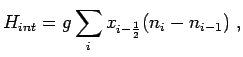(4.39)

whereis the lattice displacement. The last two parts of the Hamiltonian are once again diagonalized via the unitary operatorwhere(4.40)

Using the expansion of Eq. 4.29 we find for the transformed phonon and electron annihilation operators: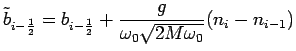(4.41)(4.42)

Substituting these transformed operators we find in the new basis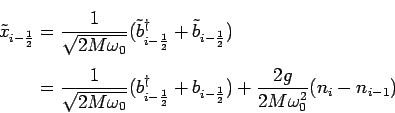(4.43)

and for the breathing-Hamiltonian(4.44)(4.45)

where(4.46)

In the case of a system only containing a single electron the density terms contained in Eq. 4.44 simplify, yielding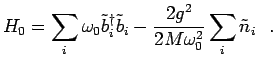(4.47)

Hence, the lattice deformation energy is found to be(4.48)

In contrast to the H model (Eq. 4.37) we incur an additional factor of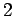due to a coupling with two neighboring oscillators. For higher dimensionality the number of neighboring oscillators is given by the coordination number, thus replacing the factorin Eq. 4.48 with.

We thus define the dimensionless coupling constant for the electron-breathing-phonon interaction(4.49)

This definition is identical to that found for the Holstein polaron besides the overall factor of.

1.0Next: Bibliography Up: 4.6 APPENDIX - Effective Previous: 4.6.1 Holstein
© Cyrill Slezak